Anzeige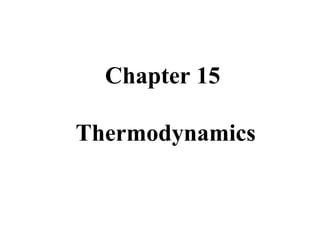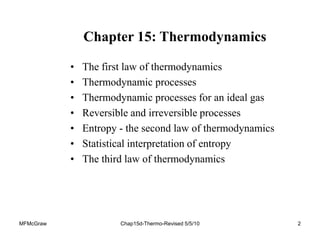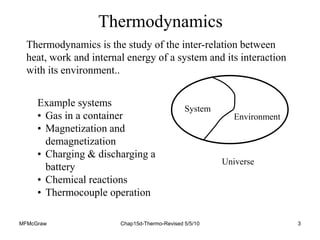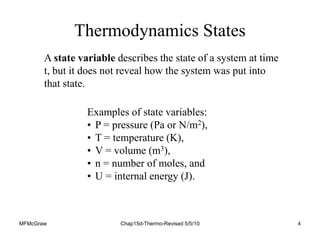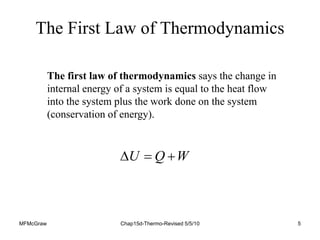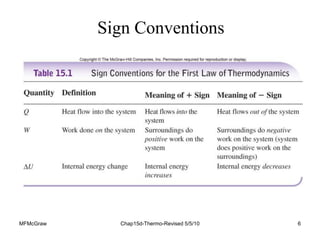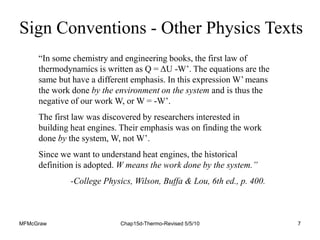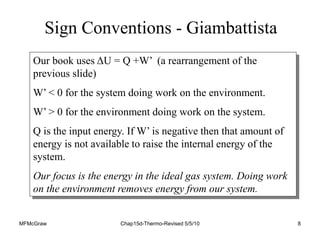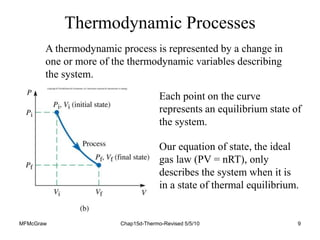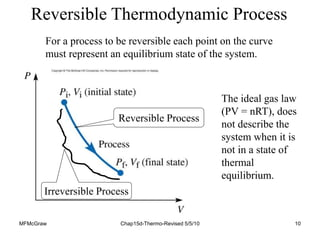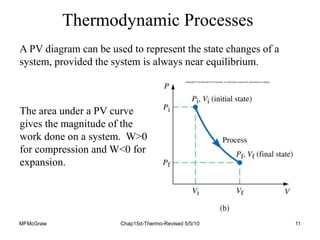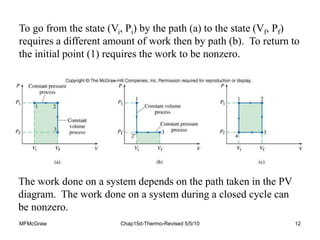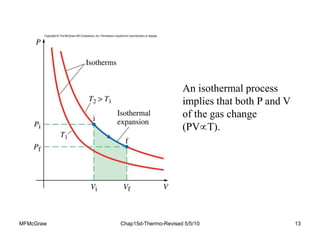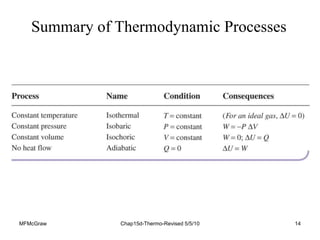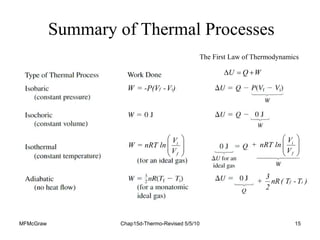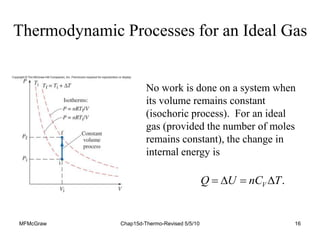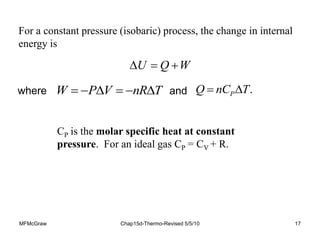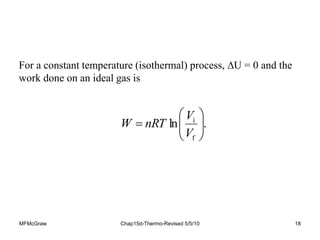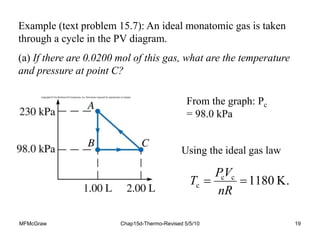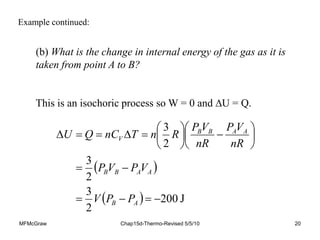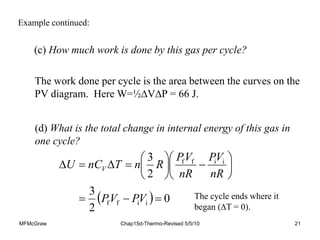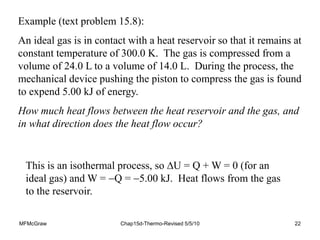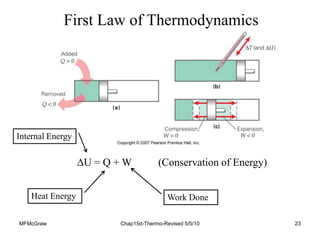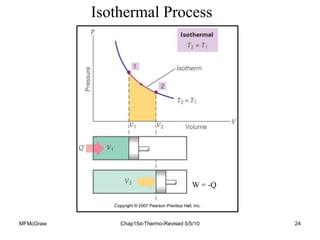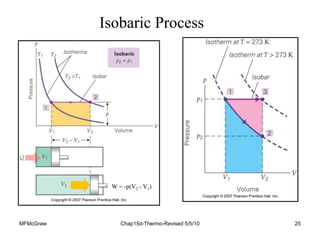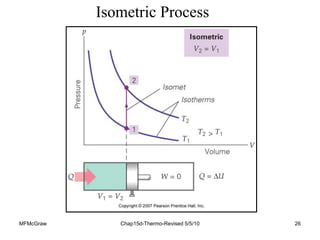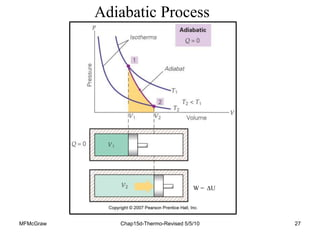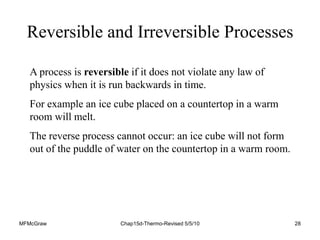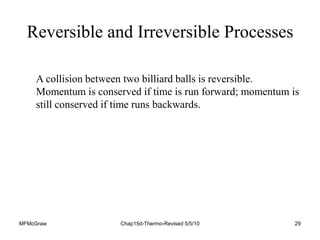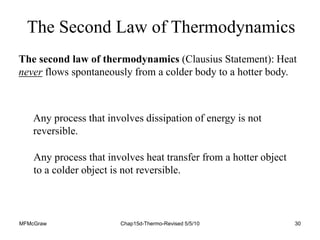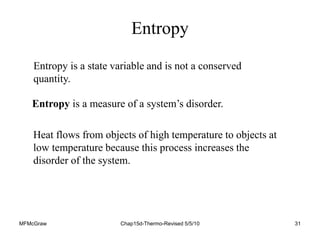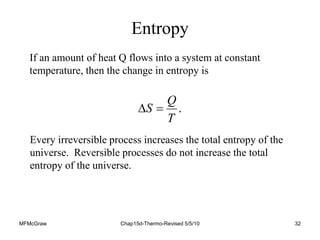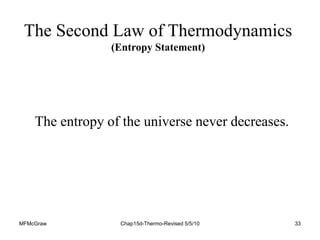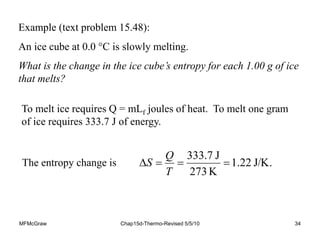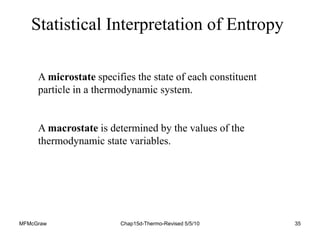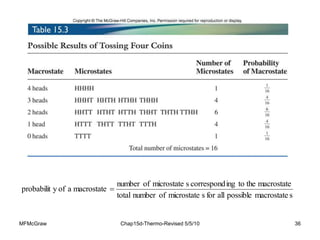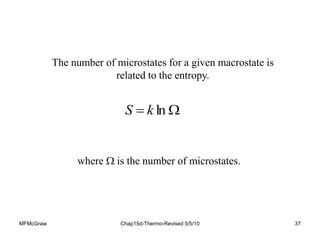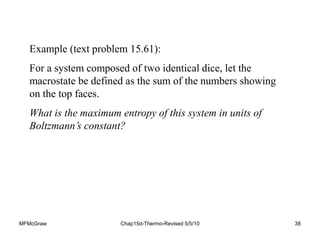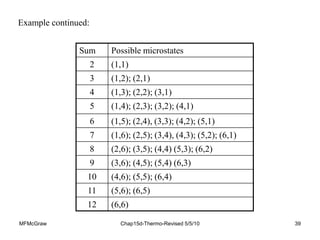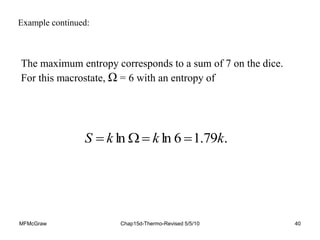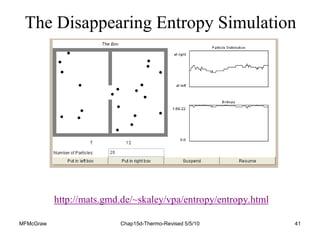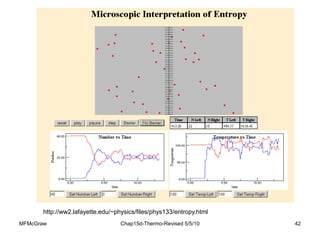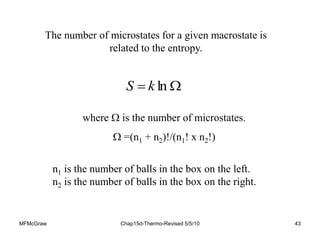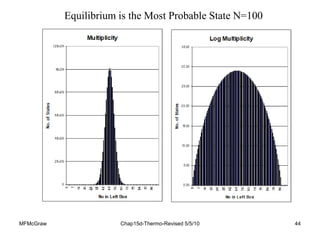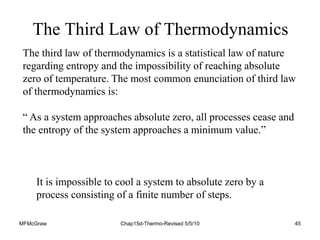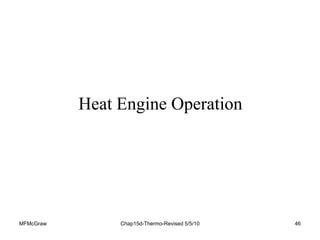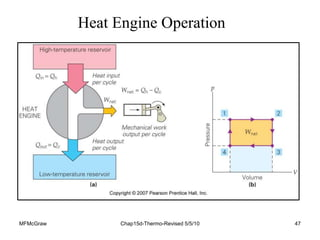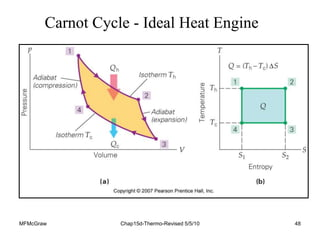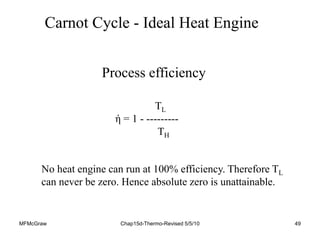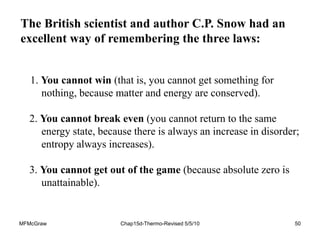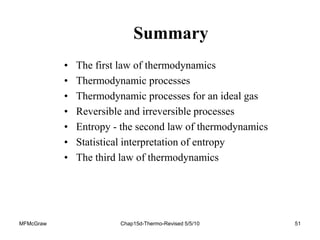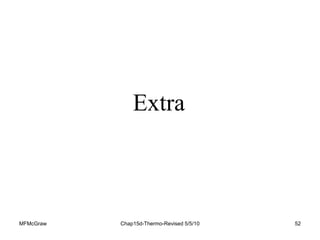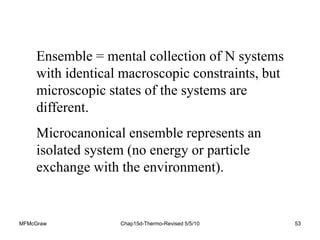1 von 53
Anzeige

### Ch_15d-WDYEA.ppt

1. Chapter 15 Thermodynamics
2. MFMcGraw Chap15d-Thermo-Revised 5/5/10 2 Chapter 15: Thermodynamics • The first law of thermodynamics • Thermodynamic processes • Thermodynamic processes for an ideal gas • Reversible and irreversible processes • Entropy - the second law of thermodynamics • Statistical interpretation of entropy • The third law of thermodynamics
3. MFMcGraw Chap15d-Thermo-Revised 5/5/10 3 Thermodynamics Example systems • Gas in a container • Magnetization and demagnetization • Charging & discharging a battery • Chemical reactions • Thermocouple operation System Environment Universe Thermodynamics is the study of the inter-relation between heat, work and internal energy of a system and its interaction with its environment..
4. MFMcGraw Chap15d-Thermo-Revised 5/5/10 4 Thermodynamics States Examples of state variables: • P = pressure (Pa or N/m2), • T = temperature (K), • V = volume (m3), • n = number of moles, and • U = internal energy (J). A state variable describes the state of a system at time t, but it does not reveal how the system was put into that state.
5. MFMcGraw Chap15d-Thermo-Revised 5/5/10 5 The First Law of Thermodynamics The first law of thermodynamics says the change in internal energy of a system is equal to the heat flow into the system plus the work done on the system (conservation of energy). W Q U   
6. MFMcGraw Chap15d-Thermo-Revised 5/5/10 6 Sign Conventions
7. MFMcGraw Chap15d-Thermo-Revised 5/5/10 7 “In some chemistry and engineering books, the first law of thermodynamics is written as Q = ΔU -W’. The equations are the same but have a different emphasis. In this expression W’ means the work done by the environment on the system and is thus the negative of our work W, or W = -W’. The first law was discovered by researchers interested in building heat engines. Their emphasis was on finding the work done by the system, W, not W’. Since we want to understand heat engines, the historical definition is adopted. W means the work done by the system.” -College Physics, Wilson, Buffa & Lou, 6th ed., p. 400. Sign Conventions - Other Physics Texts
8. MFMcGraw Chap15d-Thermo-Revised 5/5/10 8 Our book uses ΔU = Q +W’ (a rearrangement of the previous slide) W’ < 0 for the system doing work on the environment. W’ > 0 for the environment doing work on the system. Q is the input energy. If W’ is negative then that amount of energy is not available to raise the internal energy of the system. Our focus is the energy in the ideal gas system. Doing work on the environment removes energy from our system. Sign Conventions - Giambattista
9. MFMcGraw Chap15d-Thermo-Revised 5/5/10 9 Thermodynamic Processes A thermodynamic process is represented by a change in one or more of the thermodynamic variables describing the system. Each point on the curve represents an equilibrium state of the system. Our equation of state, the ideal gas law (PV = nRT), only describes the system when it is in a state of thermal equilibrium.
10. MFMcGraw Chap15d-Thermo-Revised 5/5/10 10 Reversible Thermodynamic Process For a process to be reversible each point on the curve must represent an equilibrium state of the system. The ideal gas law (PV = nRT), does not describe the system when it is not in a state of thermal equilibrium. Reversible Process Irreversible Process
11. MFMcGraw Chap15d-Thermo-Revised 5/5/10 11 A PV diagram can be used to represent the state changes of a system, provided the system is always near equilibrium. The area under a PV curve gives the magnitude of the work done on a system. W>0 for compression and W<0 for expansion. Thermodynamic Processes
12. MFMcGraw Chap15d-Thermo-Revised 5/5/10 12 The work done on a system depends on the path taken in the PV diagram. The work done on a system during a closed cycle can be nonzero. To go from the state (Vi, Pi) by the path (a) to the state (Vf, Pf) requires a different amount of work then by path (b). To return to the initial point (1) requires the work to be nonzero.
13. MFMcGraw Chap15d-Thermo-Revised 5/5/10 13 An isothermal process implies that both P and V of the gas change (PVT).
14. MFMcGraw Chap15d-Thermo-Revised 5/5/10 14 Summary of Thermodynamic Processes
15. MFMcGraw Chap15d-Thermo-Revised 5/5/10 15 Summary of Thermal Processes f i W = -P(V -V ) W Q U    The First Law of Thermodynamics         i f V W = nRT ln V         i f V + nRT ln V f i 3 + nR( T - T ) 2
16. MFMcGraw Chap15d-Thermo-Revised 5/5/10 16 Thermodynamic Processes for an Ideal Gas No work is done on a system when its volume remains constant (isochoric process). For an ideal gas (provided the number of moles remains constant), the change in internal energy is . T nC U Q V    
17. MFMcGraw Chap15d-Thermo-Revised 5/5/10 17 For a constant pressure (isobaric) process, the change in internal energy is W Q U    . T nC Q P  CP is the molar specific heat at constant pressure. For an ideal gas CP = CV + R. T nR V P W       where and
18. MFMcGraw Chap15d-Thermo-Revised 5/5/10 18 For a constant temperature (isothermal) process, U = 0 and the work done on an ideal gas is . ln f i          V V nRT W
19. MFMcGraw Chap15d-Thermo-Revised 5/5/10 19 Example (text problem 15.7): An ideal monatomic gas is taken through a cycle in the PV diagram. (a) If there are 0.0200 mol of this gas, what are the temperature and pressure at point C? From the graph: Pc = 98.0 kPa Using the ideal gas law K. 1180 c c c   nR V P T
20. MFMcGraw Chap15d-Thermo-Revised 5/5/10 20 Example continued: (b) What is the change in internal energy of the gas as it is taken from point A to B? This is an isochoric process so W = 0 and U = Q.     J 200 2 3 2 3 2 3                         A B A A B B A A B B V P P V V P V P nR V P nR V P R n T nC Q U
21. MFMcGraw Chap15d-Thermo-Revised 5/5/10 21 (c) How much work is done by this gas per cycle? (d) What is the total change in internal energy of this gas in one cycle? Example continued: The work done per cycle is the area between the curves on the PV diagram. Here W=½VP = 66 J.   0 2 3 2 3 i i f f i i f f                     V P V P nR V P nR V P R n T nC U V The cycle ends where it began (T = 0).
22. MFMcGraw Chap15d-Thermo-Revised 5/5/10 22 Example (text problem 15.8): An ideal gas is in contact with a heat reservoir so that it remains at constant temperature of 300.0 K. The gas is compressed from a volume of 24.0 L to a volume of 14.0 L. During the process, the mechanical device pushing the piston to compress the gas is found to expend 5.00 kJ of energy. How much heat flows between the heat reservoir and the gas, and in what direction does the heat flow occur? This is an isothermal process, so U = Q + W = 0 (for an ideal gas) and W = Q = 5.00 kJ. Heat flows from the gas to the reservoir.
23. MFMcGraw Chap15d-Thermo-Revised 5/5/10 23 First Law of Thermodynamics ΔU = Q + W (Conservation of Energy) Work Done Heat Energy Internal Energy
24. MFMcGraw Chap15d-Thermo-Revised 5/5/10 24 Isothermal Process W = -Q
25. MFMcGraw Chap15d-Thermo-Revised 5/5/10 25 Isobaric Process W = -p(V2 - V1)
26. MFMcGraw Chap15d-Thermo-Revised 5/5/10 26 Isometric Process
27. MFMcGraw Chap15d-Thermo-Revised 5/5/10 27 Adiabatic Process W = ΔU
28. MFMcGraw Chap15d-Thermo-Revised 5/5/10 28 Reversible and Irreversible Processes A process is reversible if it does not violate any law of physics when it is run backwards in time. For example an ice cube placed on a countertop in a warm room will melt. The reverse process cannot occur: an ice cube will not form out of the puddle of water on the countertop in a warm room.
29. MFMcGraw Chap15d-Thermo-Revised 5/5/10 29 A collision between two billiard balls is reversible. Momentum is conserved if time is run forward; momentum is still conserved if time runs backwards. Reversible and Irreversible Processes
30. MFMcGraw Chap15d-Thermo-Revised 5/5/10 30 Any process that involves dissipation of energy is not reversible. Any process that involves heat transfer from a hotter object to a colder object is not reversible. The second law of thermodynamics (Clausius Statement): Heat never flows spontaneously from a colder body to a hotter body. The Second Law of Thermodynamics
31. MFMcGraw Chap15d-Thermo-Revised 5/5/10 31 Entropy Heat flows from objects of high temperature to objects at low temperature because this process increases the disorder of the system. Entropy is a state variable and is not a conserved quantity. Entropy is a measure of a system’s disorder.
32. MFMcGraw Chap15d-Thermo-Revised 5/5/10 32 If an amount of heat Q flows into a system at constant temperature, then the change in entropy is . T Q S   Every irreversible process increases the total entropy of the universe. Reversible processes do not increase the total entropy of the universe. Entropy
33. MFMcGraw Chap15d-Thermo-Revised 5/5/10 33 The entropy of the universe never decreases. The Second Law of Thermodynamics (Entropy Statement)
34. MFMcGraw Chap15d-Thermo-Revised 5/5/10 34 Example (text problem 15.48): An ice cube at 0.0 C is slowly melting. What is the change in the ice cube’s entropy for each 1.00 g of ice that melts? To melt ice requires Q = mLf joules of heat. To melt one gram of ice requires 333.7 J of energy. J/K. 22 . 1 K 273 J 7 . 333     T Q S The entropy change is
35. MFMcGraw Chap15d-Thermo-Revised 5/5/10 35 A microstate specifies the state of each constituent particle in a thermodynamic system. A macrostate is determined by the values of the thermodynamic state variables. Statistical Interpretation of Entropy
36. MFMcGraw Chap15d-Thermo-Revised 5/5/10 36 s macrostate possible all for s microstate of number total macrostate the to ing correspond s microstate of number macrostate a of y probabilit 
37. MFMcGraw Chap15d-Thermo-Revised 5/5/10 37 The number of microstates for a given macrostate is related to the entropy.   ln k S where  is the number of microstates.
38. MFMcGraw Chap15d-Thermo-Revised 5/5/10 38 Example (text problem 15.61): For a system composed of two identical dice, let the macrostate be defined as the sum of the numbers showing on the top faces. What is the maximum entropy of this system in units of Boltzmann’s constant?
39. MFMcGraw Chap15d-Thermo-Revised 5/5/10 39 Sum Possible microstates 2 (1,1) 3 (1,2); (2,1) 4 (1,3); (2,2); (3,1) 5 (1,4); (2,3); (3,2); (4,1) 6 (1,5); (2,4), (3,3); (4,2); (5,1) 7 (1,6); (2,5); (3,4), (4,3); (5,2); (6,1) 8 (2,6); (3,5); (4,4) (5,3); (6,2) 9 (3,6); (4,5); (5,4) (6,3) 10 (4,6); (5,5); (6,4) 11 (5,6); (6,5) 12 (6,6) Example continued:
40. MFMcGraw Chap15d-Thermo-Revised 5/5/10 40 . 79 . 1 6 ln ln k k k S     Example continued: The maximum entropy corresponds to a sum of 7 on the dice. For this macrostate, Ω = 6 with an entropy of
41. MFMcGraw Chap15d-Thermo-Revised 5/5/10 41 http://mats.gmd.de/~skaley/vpa/entropy/entropy.html The Disappearing Entropy Simulation
42. MFMcGraw Chap15d-Thermo-Revised 5/5/10 42 http://ww2.lafayette.edu/~physics/files/phys133/entropy.html
43. MFMcGraw Chap15d-Thermo-Revised 5/5/10 43 The number of microstates for a given macrostate is related to the entropy.   ln k S where  is the number of microstates.  =(n1 + n2)!/(n1! x n2!) n1 is the number of balls in the box on the left. n2 is the number of balls in the box on the right.
44. MFMcGraw Chap15d-Thermo-Revised 5/5/10 44 Equilibrium is the Most Probable State N=100
45. MFMcGraw Chap15d-Thermo-Revised 5/5/10 45 The Third Law of Thermodynamics It is impossible to cool a system to absolute zero by a process consisting of a finite number of steps. The third law of thermodynamics is a statistical law of nature regarding entropy and the impossibility of reaching absolute zero of temperature. The most common enunciation of third law of thermodynamics is: “ As a system approaches absolute zero, all processes cease and the entropy of the system approaches a minimum value.”
46. MFMcGraw Chap15d-Thermo-Revised 5/5/10 46 Heat Engine Operation
47. MFMcGraw Chap15d-Thermo-Revised 5/5/10 47 Heat Engine Operation
48. MFMcGraw Chap15d-Thermo-Revised 5/5/10 48 Carnot Cycle - Ideal Heat Engine
49. MFMcGraw Chap15d-Thermo-Revised 5/5/10 49 Carnot Cycle - Ideal Heat Engine TL ή = 1 - --------- TH Process efficiency No heat engine can run at 100% efficiency. Therefore TL can never be zero. Hence absolute zero is unattainable.
50. MFMcGraw Chap15d-Thermo-Revised 5/5/10 50 1. You cannot win (that is, you cannot get something for nothing, because matter and energy are conserved). 2. You cannot break even (you cannot return to the same energy state, because there is always an increase in disorder; entropy always increases). 3. You cannot get out of the game (because absolute zero is unattainable). The British scientist and author C.P. Snow had an excellent way of remembering the three laws:
51. MFMcGraw Chap15d-Thermo-Revised 5/5/10 51 Summary • The first law of thermodynamics • Thermodynamic processes • Thermodynamic processes for an ideal gas • Reversible and irreversible processes • Entropy - the second law of thermodynamics • Statistical interpretation of entropy • The third law of thermodynamics
52. MFMcGraw Chap15d-Thermo-Revised 5/5/10 52 Extra
53. MFMcGraw Chap15d-Thermo-Revised 5/5/10 53 Ensemble = mental collection of N systems with identical macroscopic constraints, but microscopic states of the systems are different. Microcanonical ensemble represents an isolated system (no energy or particle exchange with the environment).
Anzeige